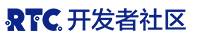# 【征文】基于OpenCV+QT开发超实用的视频编辑器

1. 视频画面添加水印；
2. 视频画面亮度调整；
3. 视频画面对比度调整；
4. 视频画面旋转；
5. 视频画面镜像；
6. 视频尺寸调整；
7. 视频图像模糊；
8. 两路视频融合。

## 3. OpenCV实战

### 3.1 OpenCV 环境搭建

``````git clone https://github.com/opencv/opencv.git
cd opencv
mkdir build
cd build
cmake -D CMAKE_BUILD_TYPE=RELEASE -D CMAKE_INSTALL_PREFIX=/usr/local/opencv3 -D BUILD_opencv_world=ON -D WITH_GSTREAMER=OFF -D OPENCV_ENABLE_NONFREE=ON ..
make -j8
sudo make install
``````

### 3.2 OpenCV核心类型Mat介绍

Mat类是Opencv中储存图像的一种数据结构。Mat类可以看做是存放矩阵的容器，他包含了两部分，分别是用来存放图片信息的信息头，和一个指向图片储存矩阵的指针。信息头往往占用空间比较小，而且各个图片之间的信息头是完全独立的。而图片储存矩阵往往占用较大的空间，并且可以多个图片的矩阵指针指向同一个内存空间。下面主要减少利用Mat创建矩阵。

#### 3.2.1 利用Mat类的构造函数创建矩阵

Mat类有很多构造函数可以用来创建矩阵结构，并且给与赋值，这里距离介绍一种，其函数定义为

`````` Mat(int rows, int cols, int type, const Scalar& s);
``````

• 第一个参数表示矩阵的行数
• 第二个参数表示矩阵的列数
• 第三个参数表示矩阵储存数据的类型,具有格式 CV_[位数] [有无符号] [数据类型] [通道数]，如`CV_8UC3 `表示存储数据为8位无符号char类型，并且具有三个通道
• 第四个参数是一种向量类型的变量，能够给予矩阵赋予初值，这个向量最多有四个维度。

#### 3.2.2 利用成员函数create创建矩阵

``````Mat m;
m.create(3, 3, CV_8UC3);
``````

### 3.3 OpenCV图像处理实战

``````#include <opencv2/imgcodecs.hpp>
#include <opencv2/imgproc.hpp>
#include <opencv2/highgui.hpp>
#include <iostream>

using namespace cv;
cv::Mat src1, src2;
cv::Mat dest; //src1.copyTo(dest);
``````

#### 3.3.1 旋转

``````//旋转90度
rotate(dest, dest, ROTATE_90_COUNTERCLOCKWISE);
//旋转180度
rotate(dest, dest, ROTATE_180);
``````

#### 3.3.2 翻转

``````//左右上下翻转
flip(dest, dest, -1);
//上下翻转
flip(dest, dest, 1);
//左右翻转
flip(dest, dest, 0);
``````

#### 3.3.3 修改大小

``````cv::resize(dest, dest, Size(width, height));
``````

#### 3.3.4 裁剪

``````dest = dest(Rect(x, y, w, h));
``````

#### 3.3.5 灰度

``````cvtColor(dest,dest, COLOR_BGR2GRAY);
``````

#### 3.3.6 混合

``````addWeighted(src2, a, dest, 1-a,0,dest);
``````

### 3.4 OpenCV视频IO接口

#### 3.4.1 打开视频源

``````VideoCapture cap1;
bool ret = cap1.open(file);
//获取帧率
fps = cap1.get(CAP_PROP_FPS);
//获取视频宽度
width = cap1.get(CAP_PROP_FRAME_WIDTH);
//获取视频高度
height = cap1.get(CAP_PROP_FRAME_HEIGHT);
``````

#### 3.4.1 读取视频帧

``````Mat mat1;
``````

## 4. QT实战

### 4.1 环境搭建

``````DEFINES += QT_MULTIMEDIA_LIB QT_WIDGETS_LIB

LIBS += -L"/usr/local/opencv3/lib" \
-lopencv_core \
-lopencv_highgui  \
-lopencv_imgproc   \
-lopencv_ml \
-lopencv_objdetect \
-lopencv_video \
-lopencv_dnn \
-lopencv_imgcodecs \
-lopencv_shape \
-lopencv_videoio \
``````

## 4.2 绘制视频

``````QImage img;
void CustomQOpenGLWidget::SetImage(cv::Mat mat){
QImage::Format fmt = QImage::Format_RGB888;
int pixSize = 3;
if(mat.type() == CV_8UC1){
fmt = QImage::Format_Grayscale8;
pixSize = 1;
}
if(img.isNull() || img.format() != fmt){
delete img.bits();
uchar *buf = new uchar[width()*height() * pixSize];
img = QImage(buf, width(), height(), fmt);
}
Mat des;
cv::resize(mat, des, Size(img.size().width(), img.size().height()));
if(pixSize > 1){
cv::cvtColor(des, des, COLOR_BGR2RGB);
}
memcpy(img.bits(), des.data, des.rows*des.rows*des.elemSize());
update();
}
void CustomQOpenGLWidget::paintEvent(QPaintEvent *e){
QPainter p;
p.begin(this);
p.drawImage(QPoint(0, 0),img);
p.end();
}
``````